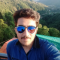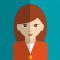## How to manually add a legend color and legend font size on a plotly figure in Python?Updated on 12-May-2023 16:06:12
This tutorial will explain how to manually add the legend text size and color on a Plotly figure using Python. By the end of this tutorial, you will be able to create interactive graphs and charts with the help of the potent Python data visualization package, Plotly. Plot development must include a legend that aids viewers in comprehending the information. However, not all situations will be accommodated by Plotly's default legend settings. This article will discuss how to manually apply legend colors and font sizes to a Plotly figure in Python. Syntax Plotly's update_layout() method and the legend_font_color and legend_font_size ... Read More

## How to create a Cumulative Histogram in Plotly?Updated on 20-Apr-2023 14:07:09
A cumulative histogram is a type of histogram that shows the cumulative distribution function (CDF) of a dataset. The CDF represents the probability that a random observation from the dataset will be less than or equal to a certain value. Cumulative histograms are useful when you want to compare the distribution of two or more datasets or when you want to visualize the proportion of data points that fall below a certain threshold. Plotly is a Python library for creating interactive and publication-quality visualizations. It is built on top of the D3.js visualization library and provides a wide range ... Read More

## How to display a variable as tooltip in ggplotly using R language?Updated on 26-Oct-2022 11:58:52
R is a programming language for statistical computing and graphics. ggplotly() is a function used to convert static plots to web-based plots. ggplotly() returns a Plotly object. In this tutorial, we will see how to display a variable as tooltip in ggplotly using R language. Here, we will use the aes() function that is used for aesthetic mapping between visual cue and a variable. It contains the following arguments: position (X and Y axes), color, fill, shape, line type, and size. To set the tooltip text, we will use the ggplotly(tooltip = " ") method. Follow the steps ... Read More

## How to show multiple ggplot2 plots with Plotly using R?Updated on 26-Oct-2022 11:56:55
R is a programming language for statistical computing and graphics. ggplotly() is a function used to convert static plots to web-based plots. ggplotly() returns a Plotly object. In this tutorial, we will see how to show multiple ggplot2 plots with Plotly using R. Here, we will use the aes() function that is used for aesthetic mapping between visual cue and a variable. It contains the following arguments: position (X and Y axes), color, fill, shape, line type, and size. To display multiple ggplot2 plots, we will use the facet_grid() function. Follow the steps given below to show multiple ... Read More

## How to remove option bar from ggplotly using R?Updated on 26-Oct-2022 11:55:03
R is a programming language for statistical computing and graphics. ggplotly() is a function that is used to convert a static plot to an interactive web-based version. ggplotly() returns a Plotly object. In this tutorial, we will see how to remove the option bar from ggplotly using R. Here, we will use the aes() function that is used for aesthetic mapping between visual cue and a variable. It contains the following arguments: position (X and Y axes), color, fill, shape, line type, and size. To remove the option bar from ggplotly, we will set "config(displayModeBar = FALSE)". Follow ... Read More

## How to format mouse over labels using ggplotly in R?Updated on 26-Oct-2022 11:52:04
R is a programming language for statistical computing and graphics. ggplotly() is a function that is used to convert a static plot to an interactive web-based version. ggplotly() returns a Plotly object. In this tutorial, we will see how to format mouse over labels using ggplotly in R. Here, we will use the aes() function that is used for aesthetic mapping between visual cue and a variable. It contains the following arguments: position (X and Y axes), color, fill, shape, line type, and size. In addition, we will use geom_line() function to set the color and the ggplotly(tooltip="") function ... Read More

## How to plot on secondary Y-Axis with Python Plotly?Updated on 26-Oct-2022 11:44:38
Plotly is an open-source, interactive, and browser-based charting library for Python. Python users can use Plotly to generate different types of charts including scientific charts, 3D graphs, statistical charts, financial charts, etc. In this tutorial, we will show how you can use Plotly to plot data on the secondary Y-Axis. Here we will use the plotly.graph_objects module to generate figures. It contains a lot of methods to customize the charts and render them into HTML format. We will plot two bar charts using the add_trace() method and then use the update_layout() method to set a property with dict arguments. Follow ... Read More

## How to add multiple text labels from DataFrame columns in Python Plotly?Updated on 26-Oct-2022 11:42:49
Plotly is an open-source plotting library in Python that can generate several different types of charts. Python users can use Plotly to create interactive web-based visualizations. In this tutorial, we will see how you can use Plotly to add multiple text labels in a chart from DataFrame columns. Here, we will use the plotly.graph_objects module to generate figures. It contains a lot of methods to customize charts and render them into HTML format. Then, we will use the Scatter() method of this module to generate a scatter plot. The "line" attribute of Scatter() contains a parameter "color" that we ... Read More

## How to add multiple graphs to a Plotly Dash app on a single browser page in Python Plotly?Updated on 21-Oct-2022 09:37:33
Plotly is an open-source plotting library in Python that can generate several different types of charts. Python users can use Plotly to create interactive web-based visualizations that can be displayed in Jupyter notebooks, saved to standalone HTML files, or served as a part of web applications using Dash. Plotly can also be used in static document publishing and desktop editors such as PyCharm and Spyder. Dash is a Python framework and it is used to create interactive web-based dashboard applications. The dash library adds all the required libraries to web based dashboard applications. In this tutorial, we will show how ... Read More

## Python Plotly – How to hide legend entries in a Plotly figure?Updated on 21-Oct-2022 09:33:23
Plotly is an open-source plotting library in Python that can generate several different types of charts. Python users can use Plotly to create interactive web-based visualizations including scientific charts, 3D graphs, statistical charts, financial charts, etc. Plotly can also be used in static document publishing and desktop editors such as PyCharm and Spyder. In this tutorial, we will show how you can use Plotly to hide legend entries for a particular field. Follow the steps given below to hide the legend entries. Step 1 Import the plotly.offline module and alias as py. import plotly.offline as py Step 2 Import ... Read More